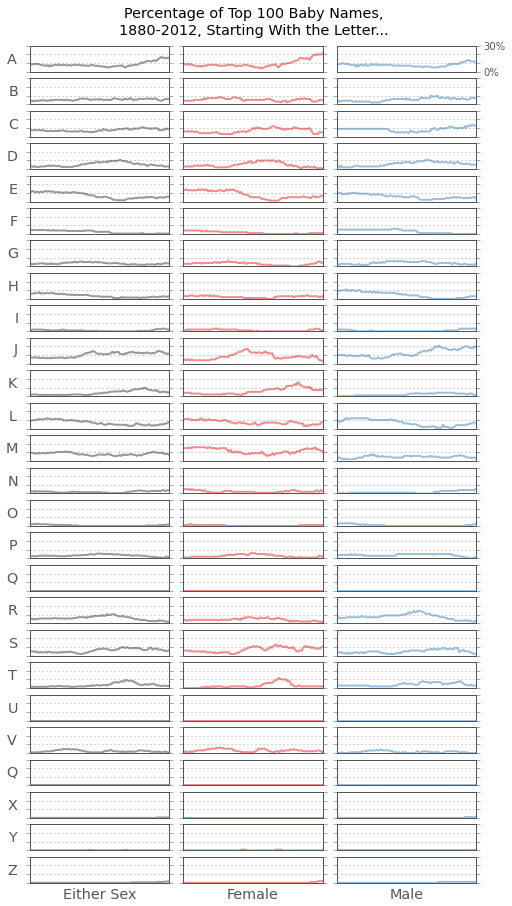# The Re-Vowelization of American Baby Names

02 Sep 2013 — Last updated: 02 Sep 2013, 10:46PM

```import pandas as pd
import matplotlib as mpl
```
```import mplstyle
import mplstyle.styles.simple
mplstyle.set(mplstyle.styles.simple)
original_colors = mplstyle.get("axes.color_cycle")
mplstyle.set({
"figure.figsize": (10, 8),
"lines.markersize": 0,
"axes.color_cycle": original_colors[:2]
})
base_style = mplstyle.get()
```

What's in a name? Vowels and consonants, mostly. But in what proportion?

The Social Security Administration keeps a list of the most popular baby names since 1880. It's a straightforward but infinitely explorable dataset, perhaps the closest thing data analysis and visualization has to a jazz standard. Let's riff on vowel usage.

```# The baby name data can be downloaded from this link:
# https://github.com/jsvine/babynames/raw/master/data/babynames.csv.bz2
#
# https://github.com/jsvine/babynames/
DATA_PATH = "/Users/jsvine/Dropbox/babynames/data/babynames.csv.bz2"

# Read the data into a pandas DataFrame
```
```# Make order of sexes explicit, and lengthen variable names
sexes = ["Female", "Male"]
names["sex"] = names["sex"].apply(lambda x: "Male" if x == "M" else "Female")
```
```# Calculate ranges useful for chart axes
years = names["year"].unique()
n_years = names["year"].max() - names["year"].min()
decades = [ 1880 + x * 10 for x in
range(n_years / 10 + 1) ]
decimals = [ float(x)/10 for x in range(11) ]
percentages = [ "%d%%" % (x * 100) for x in decimals ]
```
```# Return a new DataFrame with only the
# top `n` names per year and sex
def top_n(n):
grouped = names.groupby(["year", "sex"])
return grouped.apply(lambda x: x[:n]).reset_index(drop=True)
```
```# Helper function to set axes to sane defaults
ax.set_ylim(0, 1)
ax.set_xlim(years, years[-1] + 1)
ax.set_yticks(decimals)
ax.set_yticklabels(percentages)
ax.legend(sexes)
ax.set_xlabel("\nYear")
```
```# Add vowel data to each row
VOWELS = "AEIOU"
names["len"] = names["name"].apply(len)
names["vowels"] = names["name"].apply(lambda x: sum(let in VOWELS for let in x.upper()))
names["starts_with_vowel"] = names["name"].apply(lambda x: x in VOWELS)
```

One way to measure vowel-iness is to take the top 100 boy and girl names for each year, and calculate what proportion of their letters are vowels. Let's call this the "vowel percentage." In the chart below, you can see the vowel percentage for both sexes decrease slightly, but steadily, from 1880 through the 1950s. And you can see a gradual re-voweling since then.

```def plot_vowel_rate(df):
get_vowel_rate = lambda x: float(sum(x["vowels"])) / sum(x["len"])
grouped = df.groupby(["year", "sex"])
per_group = grouped.size()
vowel_rate = grouped.apply(get_vowel_rate).unstack()[sexes]

ax = vowel_rate.plot(grid=True, alpha=0.5)

ax.set_title("Vowels, as a Percentage of All Letters, in the\n%d Most Popular Boy/Girl Baby Names, Over Time\n" % per_group)
ax.set_ylabel("Vowel Percentage\n")
```
```plot_vowel_rate(top_n(100))
```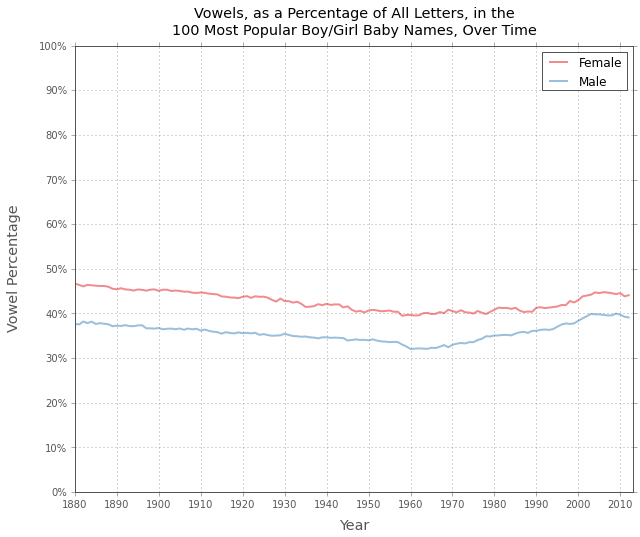Another thing you can see in this chart: female names have been consistently about five to eight percentage points more vowel-ly than male names.

But pretty much every name needs some vowels to be pronounceable. This constraint narrows the possible range inside which vowel percentage can fluctuate. The first letter of a name, however, isn't nearly so constrained. It's also typically the most distinguishing part of a name. So let's look at that.

The chart below tracks the percentage of names starting with a vowel, among the same group of names and time frame. Here you can see the de-vowelization and subsequent re-vowelization more clearly. Since 1970, the percentage of popular girl names starting with a vowel has more than tripled; for boys, the rate has more than doubled.

```def plot_first_vowel(df, **kwargs):
grouped = df.groupby(["year", "sex"])
per_group = grouped.size()
first_vowel = grouped["starts_with_vowel"].mean().unstack()

ax = first_vowel.plot(grid=True, alpha=0.5, **kwargs)
ax.set_title("Names Starting With a Vowel, as a Percentage\nof the %d Most Popular Boy/Girl Names, Over Time\n" % per_group)
ax.set_ylabel("Percentage of Names Starting With a Vowel\n")
```
```plot_first_vowel(top_n(100))
```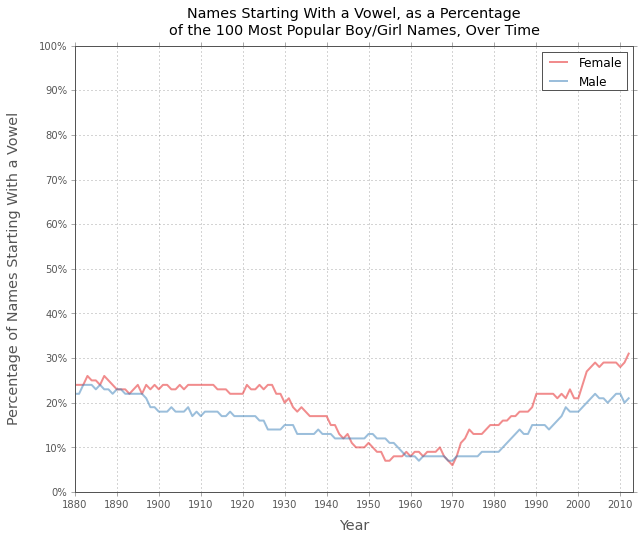The trend sharpens further among the top 20 boy and girl names. For a few years in the '40s and '50s, not a single one began with a vowel. Now, more than half of the most popular girl names names and 30% of boy names do. By this metric, the past 10 years have been the most initially-voweled on record.

```plot_first_vowel(top_n(20), drawstyle="steps")
```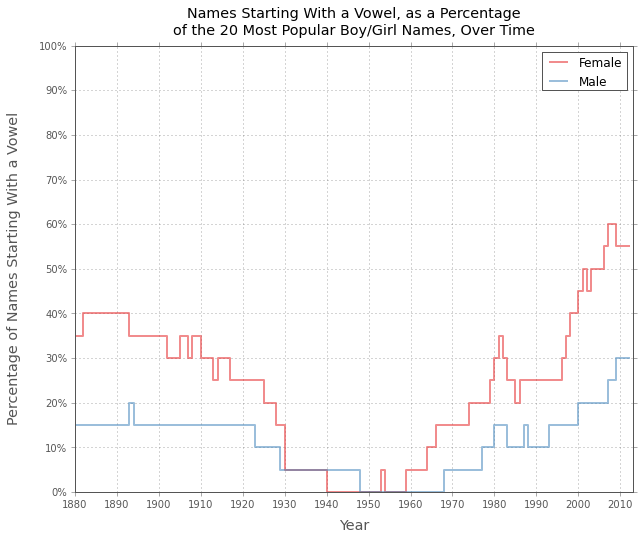You thought that was dramatic? Take a look at the next chart, which zooms in on just the top 10 boy and girl names. For more than 30 years, starting in 1930, none of the most popular boy and girl names started with a vowel. The drought for boys lasted five and a half decades.

```plot_first_vowel(top_n(10), drawstyle="steps")
```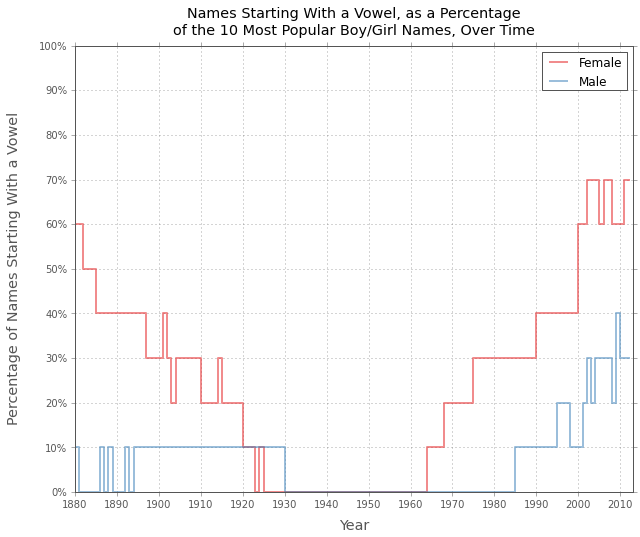```# Helper function for simple equality queries
def select(df, **kwargs):
conds = [ df[k] == v for k,v in kwargs.iteritems() ]
product = reduce(lambda m, x: m * x, conds, True)
return df[product]
```
```# Helpers for displaying Markdown
from IPython.display import HTML
from markdown import markdown
md = lambda x: HTML(markdown(x))
```
```def name_list(names):
format_name = lambda x: "__%s__" % x if x in VOWELS else x
front = u", ".join(map(format_name, names.values[:-1]))
back = ", and " + format_name(names.values[-1])
return front + back
```
```md("""
In 1945, in the middle of this vowel valley, the top 10 female baby names were: %s.

In 2012, at vowelization's latest peak, they were: %s.

In 1880, they were: %s.
""" % tuple(name_list(select(top_n(10),
year=year,
sex="Female")["name"])
for year in (1945, 2012, 1880)
))
```

In 1945, in the middle of this vowel valley, the top 10 female baby names were: Mary, Linda, Barbara, Patricia, Carol, Sandra, Nancy, Sharon, Judith, and Susan.

In 2012, at vowelization's latest peak, they were: Sophia, Emma, Isabella, Olivia, Ava, Emily, Abigail, Mia, Madison, and Elizabeth.

In 1880, they were: Mary, Anna, Emma, Elizabeth, Minnie, Margaret, Ida, Alice, Bertha, and Sarah.

```def plot_first_letter(df, let, max_y=0.3, **kwargs):
let_0 = lambda name: name == let
grouped = df.groupby("year")
per_group = grouped.size().iget(0)
first_vowel = grouped["name"].apply(lambda x: sum(map(let_0, x)) * 1. / per_group)
ax = first_vowel.plot(grid=True, **kwargs)
ax.set_ylim(0, max_y)
ax.set_yticks(decimals[:4])
ax.set_yticklabels([])
ax.set_xlim(years, years[-1])
ax.set_xticks([])
ax.set_xlabel("")
```

What letters are driving the re-vowelization of American baby names? Among the top 100 boy and girl names, "A" has done most of the work.

```def plot_first_letter_sm(n, abc = "ABCDEFGHIJKLMNOPQRSTUVQXYZ", **kwargs):
df = top_n(n)
dfs = [ df ] + [ select(df, sex=sex) for sex in sexes ]
mplstyle.set({"figure.figsize": (8, 15)})
colors = ["#333333"] + original_colors[:2]
for i in range(len(abc)):
for j in range(len(dfs)):
ax = mpl.pyplot.subplot(26, 3, i*len(dfs) + j + 1)
plot_first_letter(dfs[j], abc[i],
ax=ax,
color=(colors)[j],
alpha=0.5,
**kwargs)
ax.set_ylabel(abc[i] if not j else "", rotation=0)
top_center = mpl.pyplot.subplot(26, 3, 2)
top_center.set_title("Percentage of Top %d Baby Names,\n%d-%d, Starting With the Letter...\n" %
(n, years, years[-1]))
top_right = mpl.pyplot.subplot(26, 3, 3)
top_right.yaxis.tick_right()
top_right.set_yticklabels([ percentages, "", "", percentages ])
last_row = (len(abc) - 1) * 3 + 1
labels = ["Either Sex"] + sexes
for i in range(len(labels)):
mpl.pyplot.subplot(26, 3, last_row + i).set_xlabel(labels[i])
mplstyle.reset(base_style)
```
```plot_first_letter_sm(100, VOWELS)
```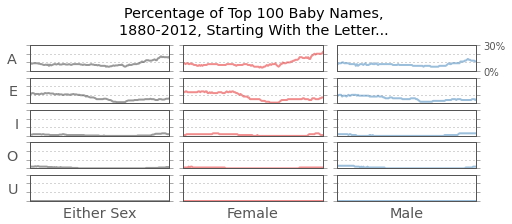Among just the 20 most popular names for each gender, we can see "E" pulling weight, too.

```plot_first_letter_sm(20, VOWELS)
```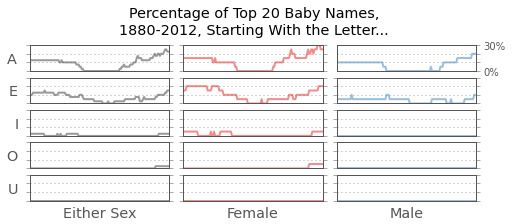Poor "U" has never once cracked the top 100 for either sex.

You can find out how your first initial has ranked over the years in the (final) chart below.

```plot_first_letter_sm(100)
```